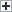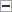## Defining an Impaired AssetWhen it comes to defining an impaired asset, its fair market value is worth less than the original cost of the asset – or, more formally, its carrying value. As a company re-evaluates its assets’ value, and when it determines there’s a discrepancy between the book or original value and the current market value, impaired assets that are lower in value are written down on the balance sheet. The business’ income statement shows a loss for the negative difference in value. Impaired assets can be Property, Plant, and Equipment (PP&E), goodwill, or fixed assets.

Making a Judgment on Asset Impairment

One more consideration to get an accurate calculation, according to generally accepted accounting principles (GAAP), is to ensure that accumulated depreciation is subtracted from the asset’s historical or original cost before assessing the difference between the fair market and carrying values. Equally as important is the GAAP recommendation for businesses to perform impairment tests annually.

Assets could be damaged physically, consumer demand may change, or legal factors could reduce its fair market value. These reasons may cause lowered projected future cash flows – lower than an asset’s current carrying value. It, therefore, requires an impairment assessment.

Illustrating With a Real-World Example

Take a business that bought a piece of equipment 24 months ago worth \$500,000 and depreciates it \$25,000 annually. Using these two figures, we can determine the equipment’s carrying value is as follows for the present year:

[(\$500,000 – (\$25,000 x 2 years)] = \$450,000

If the same type of asset (same age, usage, etc.) can be purchased on the open market but is able to be purchased for \$400,000 (market value), the asset the business owns would be considered an impaired asset.

The difference between the current market value and the carrying value is: \$450,000 – \$400,000 = \$50,000. The \$50,000 would be written down.

It’s important to note that once an asset is impaired, depreciation going forward must be recalculated based upon the new valuation figure.

Criteria to Establish Impairment

According to GAAP, businesses must begin with a recoverability test. If the initial cost of an asset (minus any depreciation or amortization) is more than the non-discount rate adjusted cash flows it’s projected to produce, the asset is considered impaired.

Assuming the asset is deemed impaired, the second part determines how much impairment exists, which is the gap between the original and market value of the asset in question. If the fair value is unspecified, the total of the discount rate adjusted future cash flows is acceptable.

Assuming the total of non-discount rate adjusted future cash flows is \$90,000 – the projected undiscounted cash flows through the next 36 months, which is lower than the estimated carry amount (or book value) of \$115,000. The recoverability test is passed, so the asset should be impaired. Based on the second step, the impairment loss will be \$25,000 (\$115,000 – \$90,000). If, however, the fair market value is unknown, the projected cash flows of \$30,000 per year for the next 36 months should be discounted to present value. This example can assume a 5 percent discount rate:

Year 1 – \$30,000 / (1+0.05) = \$30,000 / 1.05 = (\$28,571.43)

Year 2 – \$30,000 / (1+0.05)^2 = \$30,000 / (1.1025) = (\$27,210.88)

Year 3 – \$30,000 / (1+0.05)^3 = \$30,000 / (1.1576) = (\$25,915.69)

To calculate the impairment loss with an unknown fair market value: \$115,000 – (\$28,571.43 + \$27,210.88 + \$25,915.69) = \$115,000 – \$81,698.00 = \$33,302.00

Whether it’s a time of economic uncertainty or the economy is firing on full cylinders, assets can change value. Businesses that effectively navigate changing conditions are able to increase their chances of surviving or thriving amid the challenges they might face.

Disclaimer